## Design Controller Using MPC Designer

This example shows how to design a model predictive controller for a continuous stirred-tank reactor (CSTR) using MPC Designer.

### CSTR Model

The following differential equations represent the linearized model of a continuous stirred-tank reactor (CSTR) involving an exothermic reaction:

`$\frac{d{{C}^{\prime }}_{A}}{dt}={a}_{11}{{C}^{\prime }}_{A}+{a}_{12}{T}^{\prime }+{b}_{11}{{T}^{\prime }}_{c}+{b}_{12}{{C}^{\prime }}_{Ai}$`

`$\frac{d{T}^{\prime }}{dt}={a}_{21}{{C}^{\prime }}_{A}+{a}_{22}{T}^{\prime }+{b}_{21}{{T}^{\prime }}_{c}+{b}_{22}{{C}^{\prime }}_{Ai}$`

where the inputs are:

• CAi — Concentration of reagent A in the feed stream (kgmol/m3)

• Tc — Reactor coolant temperature (degrees C)

and the outputs are:

• T — Reactor temperature (degrees C)

• CA — Residual concentration of reagent A in the product stream (kgmol/m3)

The prime terms, such as CA, denote a deviation from the nominal steady-state condition at which the model has been linearized.Measurement of reagent concentrations is often difficult. For this example, assume that:

• Tc is a manipulated variable.

• CAi is an unmeasured disturbance.

• T is a measured output.

• CA is an unmeasured output.

The model can be described in state-space format:

`$\frac{dx}{dt}=Ax+Bu$`

`$y=Cx+Du$`

where,

For this example, the coolant temperature has a limited range of ±10 degrees from its nominal value and a limited rate of change of ±4 degrees per sample period.

Create a state-space model of a CSTR system.

```A = [-0.0285 -0.0014; -0.0371 -0.1476]; B = [-0.0850 0.0238; 0.0802 0.4462]; C = [0 1; 1 0]; D = zeros(2,2); CSTR = ss(A,B,C,D);```

### Import Plant and Define MPC Structure

`mpcDesigner`On the MPC Designer tab, in the Structure section, click MPC Structure.

In the Define MPC Structure By Importing dialog box, in the Select a plant model or an MPC controller table, select the `CSTR` model.

Since `CSTR` is a stable, continuous-time LTI system, MPC Designer sets the controller sample time to 0.1 Tr, where Tr is the average rise time of `CSTR`. For this example, in the Specify MPC controller sample time field, enter a sample time of `1`.

By default, all plant inputs are defined as manipulated variables and all plant outputs as measured outputs. In the Assign plant i/o channels section, assign the input and output channel indices such that:

• The first input, coolant temperature, is a manipulated variable.

• The second input, feed concentration, is an unmeasured disturbance.

• The first output, reactor temperature, is a measured output.

• The second output, reactant concentration, is an unmeasured output.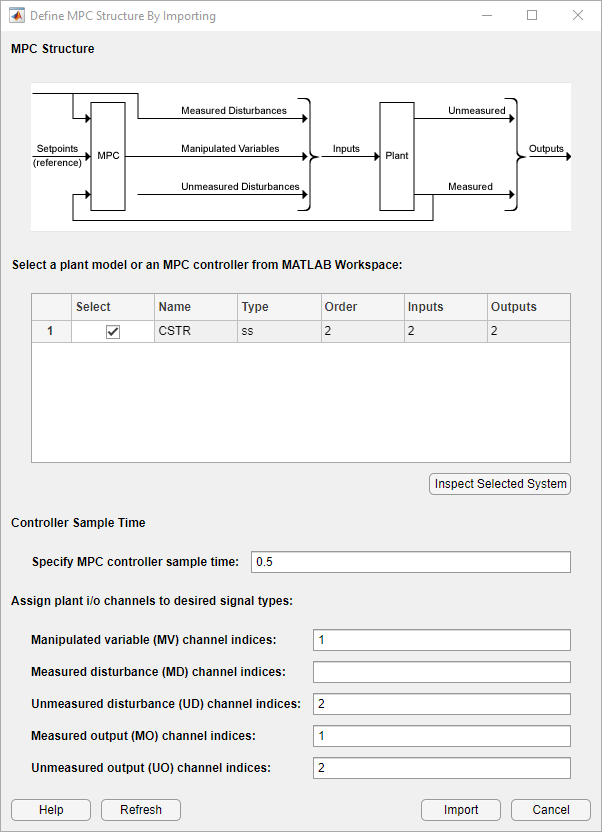Click .

The app imports the `CSTR` plant to the Data Browser. The following are also added to the Data Browser:

• `mpc1` — Default MPC controller created using `sys` as its internal model.

• `scenario1` — Default simulation scenario.

The app runs the default simulation scenario and updates the Input Response and Output Response plots.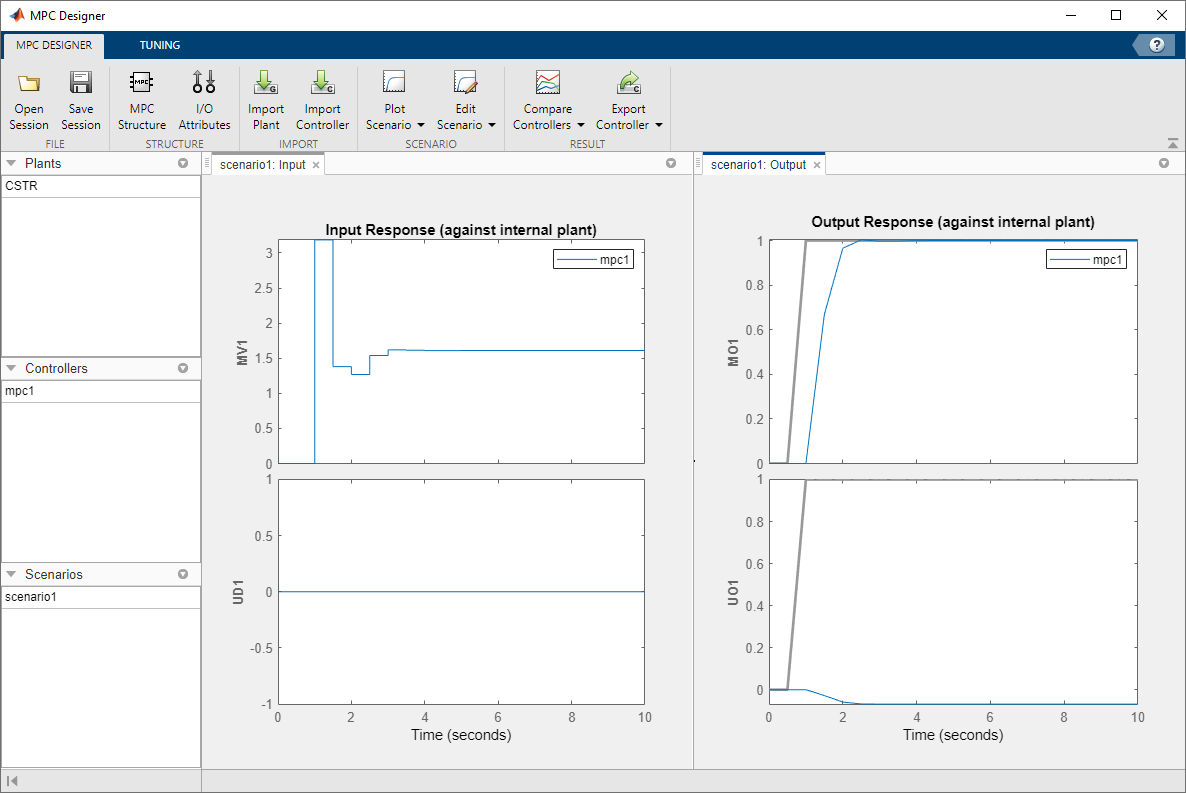Tip

To view the response plots side-by-side, on the View tab, in the Tiles section, click Left/Right.Once you define the MPC structure, you cannot change it within the current MPC Designer session. To use a different channel configuration, start a new session of the app.

### Define Input and Output Channel Attributes

On the MPC Designer tab, select I/O Attributes.

In the Input and Output Channel Specifications dialog box, in the Name column, specify a meaningful name for each input and output channel.

In the Unit column, optionally specify the units for each channel.

Since the state-space model is defined using deviations from the nominal operating point, set the Nominal Value for each input and output channel to `0`.

Keep the Scale Factor for each channel at the default value of `1`.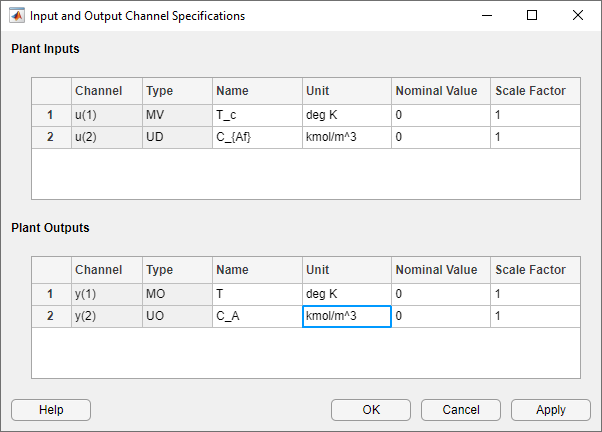Click .

The Input Response and Output Response plot labels update to reflect the new signal names and units.

### Configure Simulation Scenario

On the MPC Designer tab, in the Scenario section, click Edit Scenario > scenario1.

In the Simulation Scenario dialog box, increase the Simulation duration to `30` seconds.

In the Reference Signals table, in the first row, specify a step Size of `2` and a Time of `5`.

In the Signal column, in the second row, select a `Constant` reference to hold the concentration setpoint at its nominal value.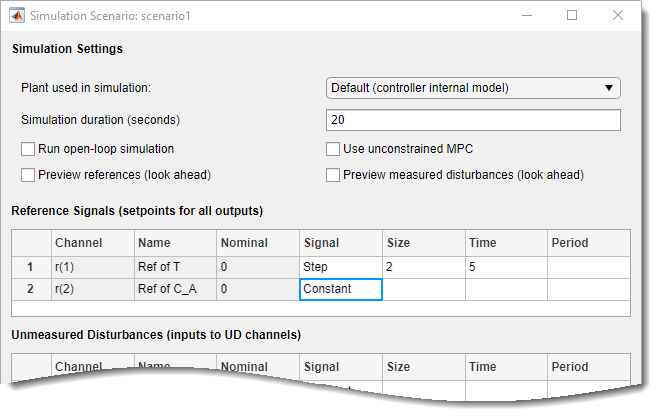The default scenario is configured to simulate a step change of `2` degrees in the reactor temperature, T, at a time of `5` seconds.

Click .

The response plots update to reflect the new simulation scenario configuration.

In the Data Browser, in the Scenarios section, click `scenario1`. Click `scenario1` a second time, and rename the scenario to `stepT`.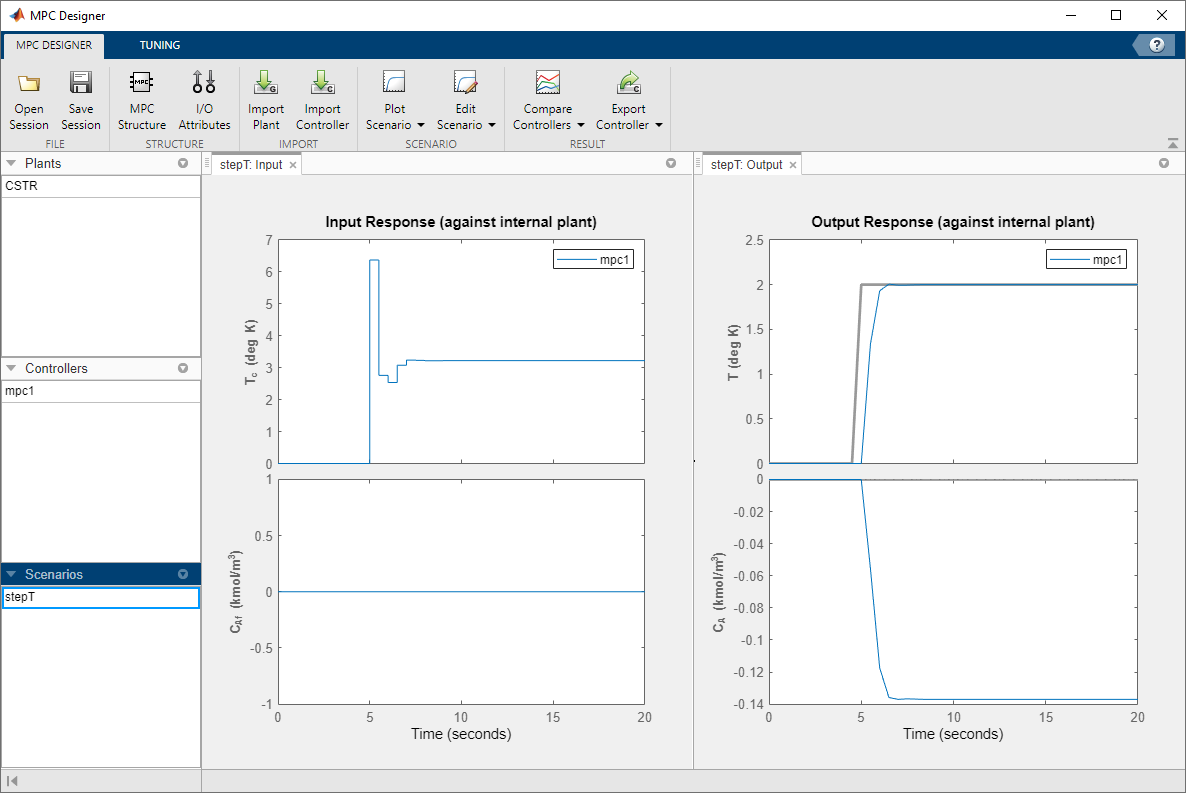### Configure Controller Horizons

On the Tuning tab, in the Horizons section, specify a Prediction horizon of `15` and a Control horizon of `3`.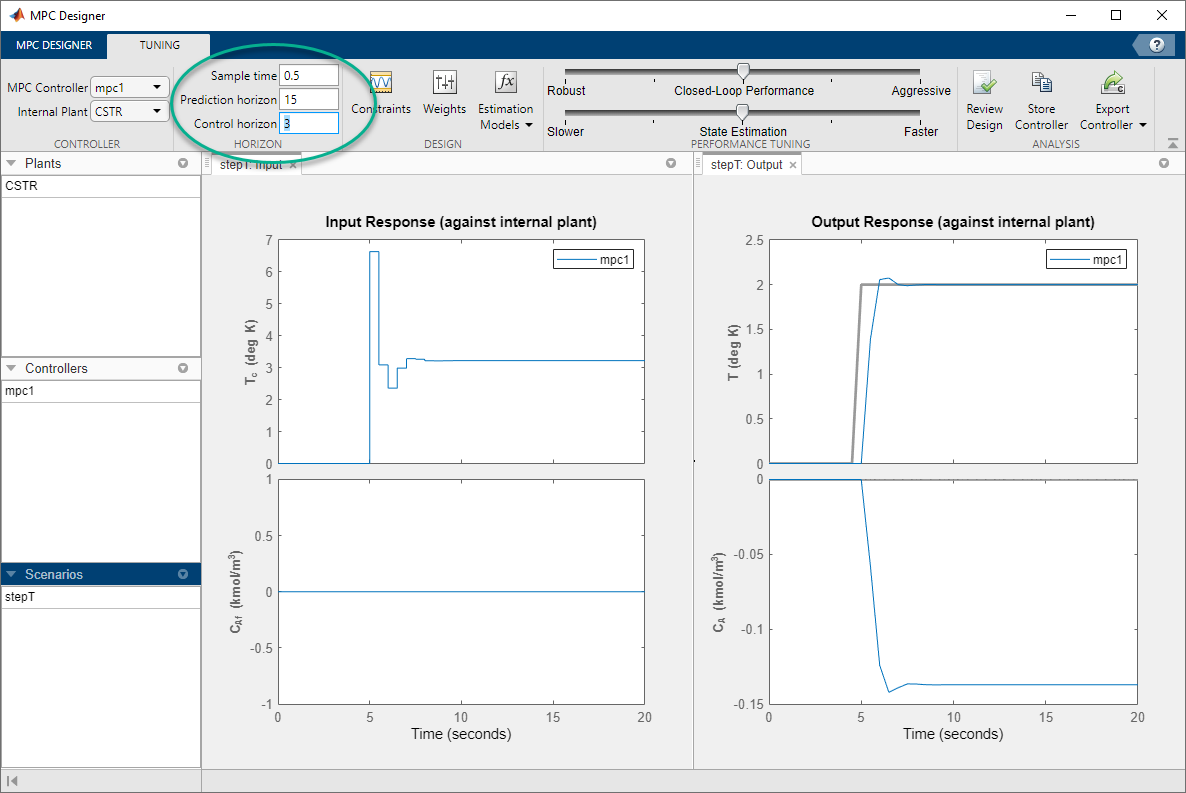The response plots update to reflect the new horizons. The Input Response plot shows that the control actions for the manipulated variable violate the required coolant temperature constraints.

### Define Input Constraints

In the Design section, click Constraints.

In the Constraints dialog box, in the Input Constraints section, enter the coolant temperature upper and lower bounds in the Min and Max columns respectively.

Specify the rate of change limits in the RateMin and RateMax columns.Click .The Input Response plot shows the constrained manipulated variable control actions. Even with the constrained rate of change, the coolant temperature rises quickly to its maximum limit within three control intervals.

### Specify Controller Tuning Weights

On the Tuning tab, in the Design section, click Weights.

In the Input Weights table, increase the manipulated variable (MV) Rate Weight to `0.3`. Increasing the MV rate weight penalizes large MV changes in the controller optimization cost function.

In the Output Weights table, keep the default Weight values. By default, all unmeasured outputs have zero weights.

Since there is only one manipulated variable, if the controller tries to hold both outputs at specific setpoints, one or both outputs will exhibit steady-state error in their responses. Since the controller ignores setpoints for outputs with zero weight, setting the concentration output weight to zero allows reactor temperature setpoint tracking with zero steady-state error.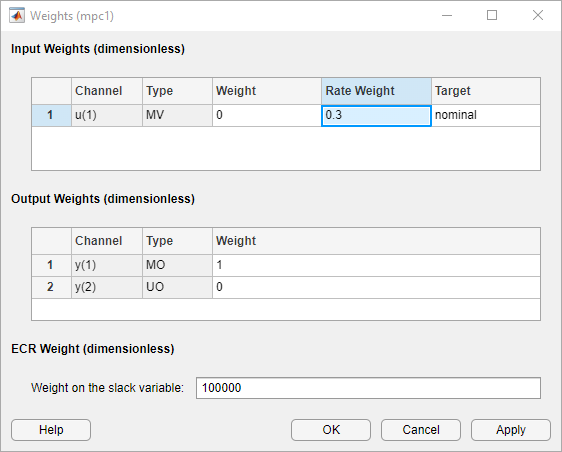Click .The Input Response plot shows the more conservative control actions, which result in a slower Output Response.

### Eliminate Output Overshoot

Suppose the application demands zero overshoot in the output response. On the Performance Tuning tab, drag the Closed-Loop Performance slider to the left until the Output Response has no overshoot. Moving this slider to the left simultaneously increases the manipulated variable rate weight of the controller and decreases the output variable weight, producing a more robust controller.When you adjust the controller tuning weights using the Closed-Loop Performance slider, MPC Designer does not change the weights you specified in the Weights dialog box. Instead, the slider controls an adjustment factor, which is used with the user-specified weights to define the actual controller weights.

This factor is `1` when the slider is centered; its value decreases as the slider moves left and increases as the slider moves right. The weighting factor multiplies the manipulated variable and output variable weights and divides the manipulated variable rate weights from the Weights dialog box.

To view the actual controller weights, export the controller to the MATLAB® workspace, and view the `Weights` property of the exported controller object.

### Test Controller Disturbance Rejection

In a process control application, disturbance rejection is often more important than setpoint tracking. Simulate the controller response to a step change in the feed concentration unmeasured disturbance.

On the MPC Designer tab, in the Scenario section, click Plot Scenario > New Scenario.

In the Simulation Scenario dialog box, set the Simulation duration to 30 seconds.

In the Unmeasured Disturbances table, in the Signal drop-down list, select `Step`.

In the Time column, specify a step time of `5` seconds.Click .

The app adds new scenario to the Data Browser and creates new corresponding Input Response and Output Response plots.

In the Data Browser, in the Scenarios section, rename `NewScenario` to `distReject`.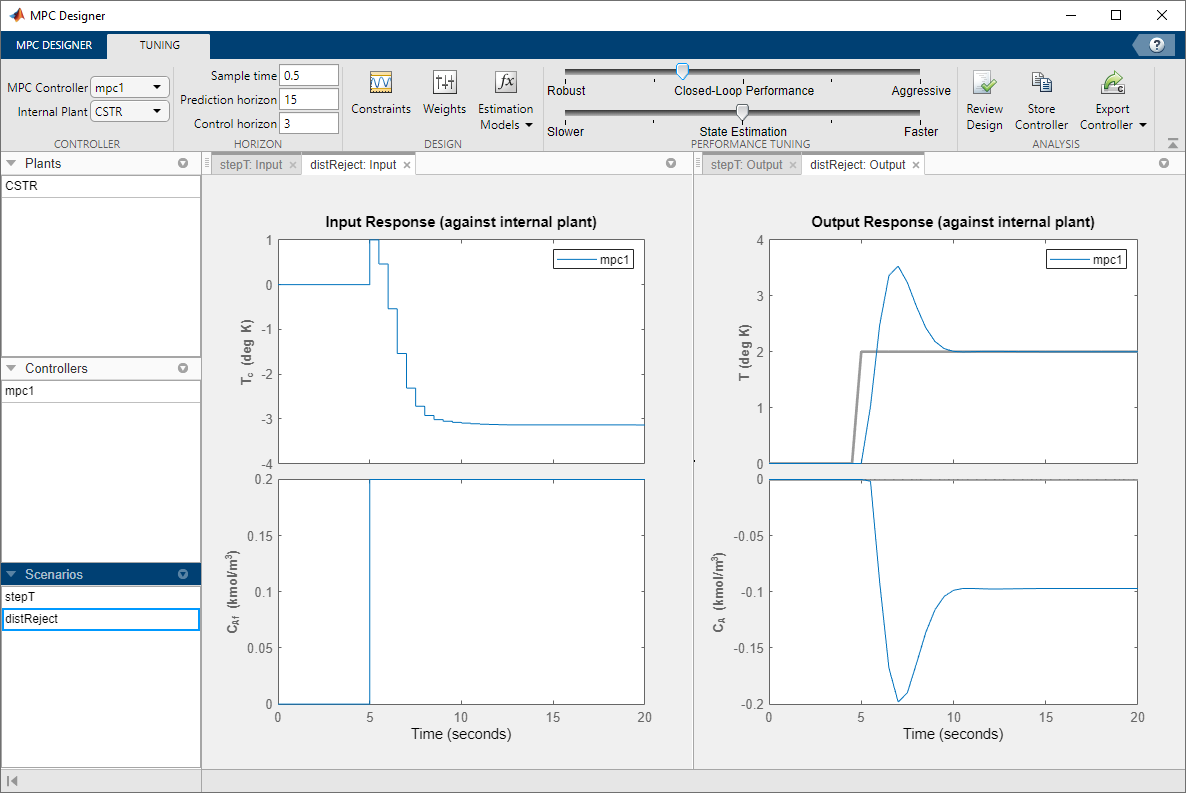In the Output Response plots, the controller returns the reactor temperature, T, to a value near its setpoint as expected. However, the required control actions cause an increase in the output concentration, CA to 6 kgmol/m3.

### Specify Concentration Output Constraint

Previously, you defined the controller tuning weights to achieve the primary control objective of tracking the reactor temperature setpoint with zero steady-state error. Doing so enables the unmeasured reactor concentration to vary freely. Suppose that unwanted reactions occur once the reactor concentration exceeds a 3 kgmol/m3. To limit the reactor concentration, specify an output constraint.

On the Tuning tab, in the Design section, click Constraints.

In the Constraints dialog box, in the Output Constraints section, the second row of the table, specify a Max unmeasured output (UO) value of `3`.

In the Output Constraints section, click .

By default, all output constraints are soft, meaning that their MinECR and MaxECR values are greater than zero. To soften the unmeasured output (UO) constraint further, increase its MaxECR value.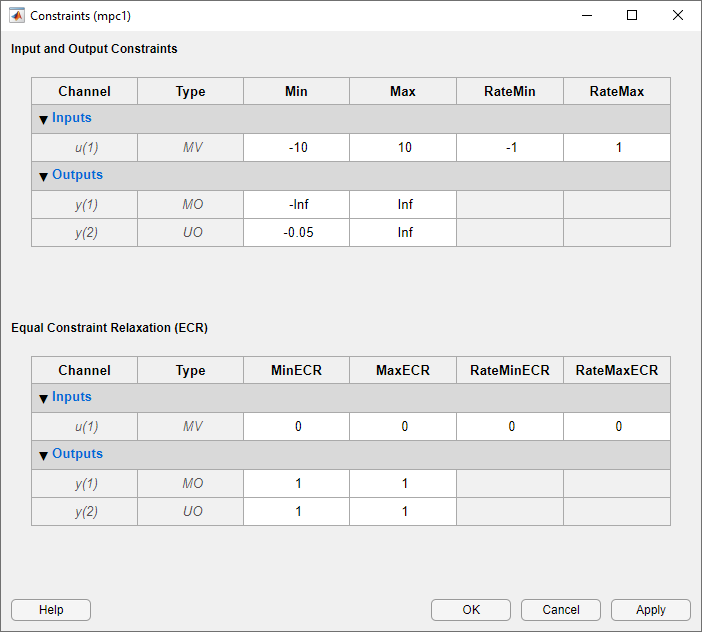Click OK.In the Output Response plots, once the reactor concentration, CA, approaches 3 kgmol/m3, the reactor temperature, T, starts to increase. Since there is only one manipulated variable, the controller makes a compromise between the two competing control objectives: Temperature control and constraint satisfaction. A softer output constraint enables the controller to sacrifice the constraint requirement more to achieve improved temperature tracking.

Since the output constraint is soft, the controller maintain adequate temperature control by allowing a small concentration constraint violation. In general, depending on your application requirements, you can experiment with different constraint settings to achieve an acceptable control objective compromise.

### Export Controller

In the Tuning tab, in the Analysis section, click Export Controllerto save the tuned controller, `mpc1`, to the MATLAB workspace.

### Delete Plants, Controllers, and Scenarios

To delete a plant, controller, or scenario, in the Data Browser, right-click the item you want to delete, and select Delete. You can also click the item and hit Delete on the keyboard.

You cannot delete the current controller. Also, you cannot delete a plant or scenario if it is the only listed plant or scenario.

If a plant is used by any controller or scenario, you cannot delete the plant.

To delete multiple plants, controllers, or scenarios, hold Shift and click each item that you want to delete.

 Seborg, D. E., T. F. Edgar, and D. A. Mellichamp, Process Dynamics and Control, 2nd Edition, Wiley, 2004, pp. 34–36 and 94–95.